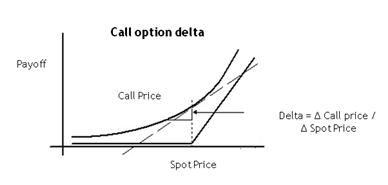# Latest content was relocated to https://bintanvictor.wordpress.com. This old blog will be shutdown soon.

## Friday, December 17, 2010

### option valuation graph - hovers above hockey stick

The vanilla European Call (or Put's) valuation graph against spot price is a _smooth_ curve slightly above the kinky hockey stick. I like to remind myself the curve depicts a "range of possibilities". The curve is a Snapshot of a bunch of what-if possibilities such as "If underlier becomes \$S my call's theoretical value is \$C".Q: since S and C axes are both in dollars, is this graph drawn in scale? Can I take a point on the smooth curve and read actual (x=100,y=20) coordinate values and interpret them as S=\$100, C=\$20?
A: yes you can. In fact the slope of the hockey stick handle is  45 degrees (representing a delta of 100% when sigma is 0.)

Let's assume strike is \$100.

For OTM, C might be \$7 when S is \$50 (below strike). C is a small fraction of S. In other words S/C represents a high leverage ratio for an OTM call (or put). C is purely time-value, since intrinsic value is \$0. Let me repeat, for OTM call (or put), leverage ratio is high because valuation is purely time-value.

The magnitude of the leverage ratio (and the valuation of the call/put) depends fundamentally on the contest between ln(S/K) vs σ√ t , as described elsewhere in this blog.

For ITM, leverage ratio is much lower and probably less meaningful. When S is \$180, C is dominated by intrinsic value of S-K = \$80. In other words, the smooth curve hovers slightly above hockey stick handle